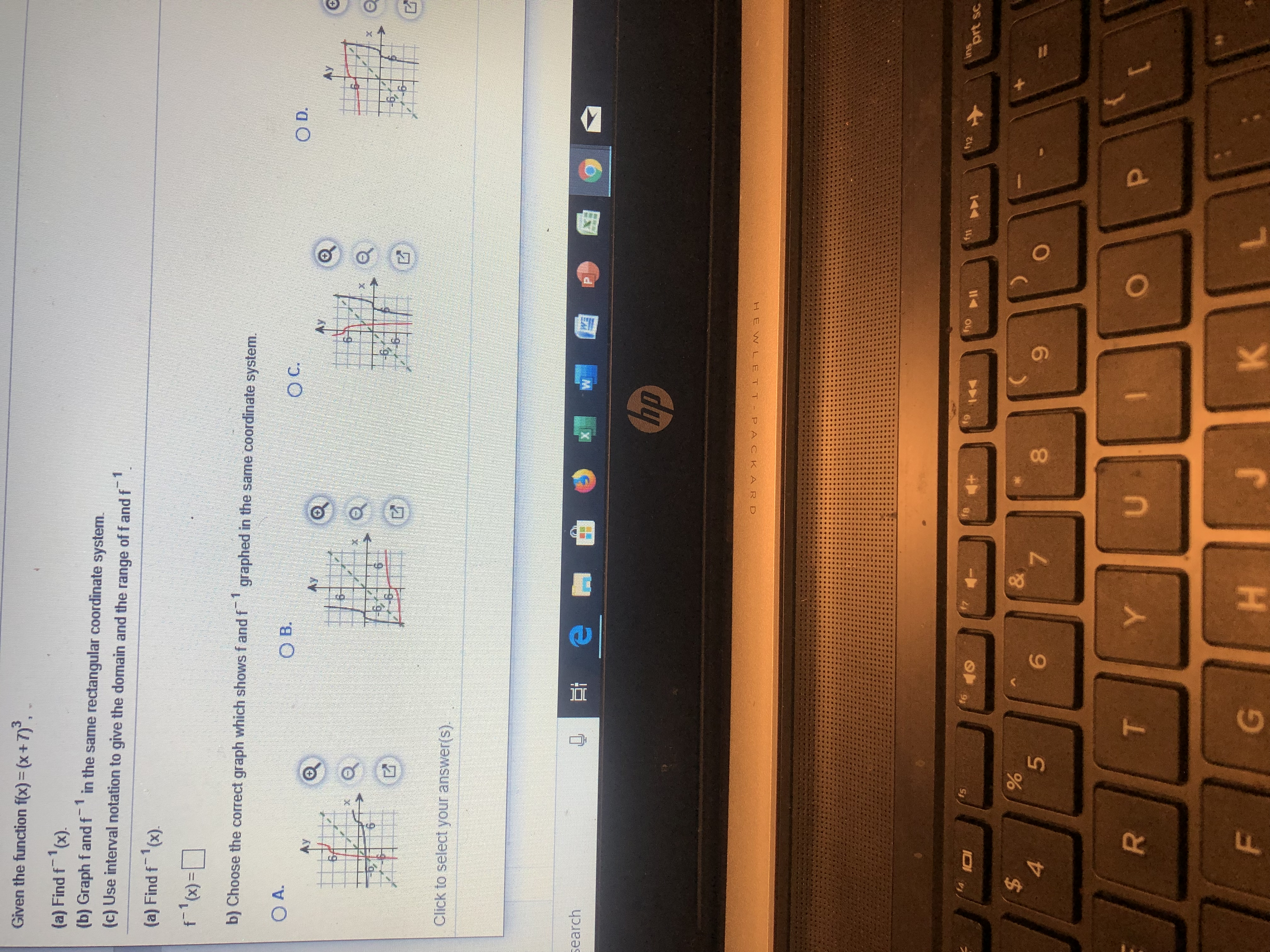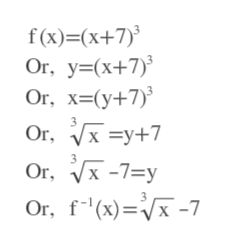# Given the function fx)- (x +7),(a) Find f (x).(b) Graph f andfin the same rectangular coordinate system.1(c) Use interval notation to give the domain and the range of f and f(a) Find f (x)f (x)1b) Choose the correct graph which shows f and f graphed in the same coordinate system.O D.O CO B.O A.Ay6h6HClick to select your answer(S)PsearchhpHEW LE T T PAC KA R Dh2f1prt scho lJ44f6fsI01&7%5\$9PUYKFHT

Question
24 viewshelp_outlineImage TranscriptioncloseGiven the function fx)- (x +7), (a) Find f (x). (b) Graph f andf in the same rectangular coordinate system. 1 (c) Use interval notation to give the domain and the range of f and f (a) Find f (x) f (x) 1 b) Choose the correct graph which shows f and f graphed in the same coordinate system. O D. O C O B. O A. Ay 6h 6H Click to select your answer(S) P search hp HEW LE T T PAC KA R D h2 f1 prt sc ho l J 44 f6 fs I01 & 7 % 5 \$ 9 P U Y K F H T fullscreen
check_circle

Step 1

We first replace f(x) by y. Then we switch x and y. And then solve for y. This new y is replaced by f^-1(x).help_outlineImage Transcriptionclosef(x) (x+7) Or, y(x+7) Or, x-(y+7) Or |x =y+7 , V-7=y Or Or, f(x)-7 fullscreen
Step 2

### Want to see the full answer?

See Solution

#### Want to see this answer and more?

Solutions are written by subject experts who are available 24/7. Questions are typically answered within 1 hour.*

See Solution
*Response times may vary by subject and question.
Tagged in

### Other